## Chapter 1Introduction

With the continued shrinking of semiconductor devices, a deeper understanding of the underlying physical processes is required in order to further improve device performance. Since it is impossible to measure all details of carrier transport on the nanometer scale, the availability of accurate theoretical descriptions is essential in order to gain insight into the physical processes by means of numerical simulations. This so-called Technology Computer Aided Design (TCAD) has become an indispensable ingredient for the development of faster, smaller and more power-efficient devices.

Quantum effects have long been negligible for charge transport, but they gain importance with each technology generation. Nevertheless, quantum effects are not considered further within this thesis, even though the theory of carrier scattering is based on a quantum mechanical foundation  .

### 1.1 Semiclassical Carrier Transport

The Boltzmann Transport Equation (BTE) is commonly considered to provide the best semiclassical description of carrier transport. Carriers are described in a classical fashion by a continuous distribution function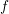, which depends on the spatial location, momentum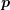and time. The carrier momentumis related to a quantum-mechanical wave number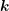by the relation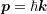, where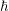is the modified Planck constant. Without going into the details of various derivations (see e.g.  ), the BTE is given by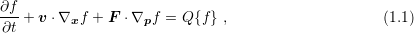where function arguments are omitted. Here,denotes the carrier velocity in dependence of the carrier momentum,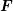refers to the electrostatic force, andis the scattering operator. A formulation based on the wavevectorrather than momentumtransforms the gradient as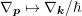.

The description of carries by means of a distribution function with respect to the spatial variable, the momentumand timeleads to a seven-dimensional problem space, which makes the direct solution of the BTE very demanding. As a consequence, simpler macroscopic models have been derived from moments of the BTE. Most noteworthy in this regard are the drift-diffusion equations presented in Sec. 1.1.1, which are obtained from the zeroth and first moment of the BTE.

While the drift-diffusion equations have been successfully employed throughout the 20th century for TCAD, the characteristic lengths of modern devices are well outside the range of validity of the model. Hence, even though variants of the drift-diffusion equations are still in use for the simulation of recent device generations, their accuracy is highly questionable and leads to poor results already in the linear regime  . More accurate macroscopic transport models have been derived based on higher moments of the Boltzmann Transport Equation (BTE). The energy transport model and the hydrodynamic model are derived from the first four moments of the BTE and presented in Sec. 1.1.3 and Sec. 1.1.2 respectively. In Sec. 1.1.4 a transport model based on the first six moments of the BTE is discussed.

Despite the good results obtained from macroscopic models, which are derived from moments of the BTE in the micron and sub-micron regime, the necessary approximations cease to hold in the deca-nanometer regime. As a consequence, a full solution of the BTE is desired. Since direct numerical methods are typically limited by memory constraints which stem from the resolution of the high-dimensional simulation domain, the method of choice is usually the stochastic Monte Carlo method, which is presented in Sec. 1.1.5.

It should be noted that each of the transport models presented in the following has to be solved self-consistently with the Poisson equation. To this end, nonlinear iteration schemes such as those detailed in Sec. 2.4 are typically employed. For the sake of brevity, self-consistency is not further addressed in the following subsections.

#### 1.1.1 The Drift-Diffusion Model

Taking the zeroth-order moment of the BTE (1.1) leads to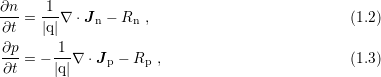whereand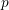denote electron and hole densities and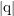is the elementary charge1 . The recombination rates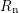and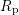refer to Auger, radiative, and Shockley-Read-Hall   processes and can be expressed in terms ofand. Multiplication of the BTE with the wave vectorand integration over the-space leads to equations for the current densities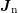and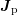: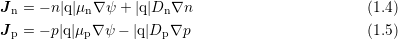Carrier mobilities are denoted by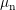and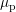, while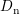and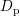are diffusion coefficients. Substitution of (1.4) and (1.5) into (1.2) and (1.3) leads to a system of two partial differential equations for the densitiesand. Equipped with suitable boundary conditions, these equations completely specify the electron and hole densities. This so-called drift-diffusion model was first derived by Van Roosbroeck in 1950  .

As a prelude to numerical stabilization schemes discussed in Sec. 4.3, a direct discretization of the drift-diffusion model by simple methods such as finite differences usually fails due to large forces inside the device. Numerical stability for the drift-diffusion equations is increased substantially by the use of upwind schemes in general, and the Scharfetter-Gummel scheme   in particular. Additionally, a discretization is usually required to conserve current, hence box integration schemes are commonly employed  .

#### 1.1.2 The Hydrodynamic Model

The consequence of taking the zeroth and the first moment of the BTE for the derivation of the drift-diffusion model only is that a spatial dependence of average carrier energies are ignored. To overcome these deficiencies, Bløtkjær derived conservation equations by taking the zeroth, the first and the second moments of the BTE  . As closure condition for the heat flux density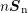, Fourier’s law is applied, which leads for electrons to the system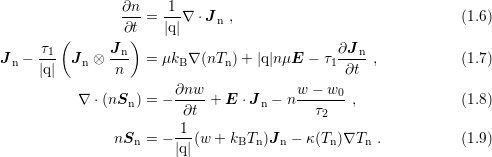The model needs to be solved for the unknown electron density, the electron temperature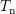and the average energy. The contribution of the drift velocity to the carrier energy is often neglected, resulting in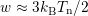. The parameters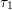and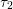stem from the first and the second moment of the scattering operator in the relaxation time approximation,refers to the thermal conductivity and is given by the Wiedemann-Franz law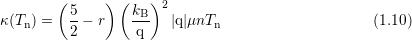with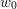denoting the average energy at equilibrium. The correction factorstems from the fact that thermal and electrical conductivity do not exclusively involve the same carriers. A similar set of equations is obtained for holes.

Equations (1.6) to (1.9) describe the full hydrodynamic model for parabolic band structures. The name stems from the similarity to the Euler equations of fluid dynamics with the addition of a heat conduction term and the collision terms. Due to its hyperbolic nature at high electron flows, the hydrodynamic model can lead to shock waves inside the device, which shows up in short-length devices or low temperatures. This leads to additional effort required for stable numerical schemes compared to the parabolic convection-diffusion type of the drift-diffusion equations.

#### 1.1.3 The Energy Transport Model

A frequent approximation to the hydrodynamic equations (1.6) to (1.9) is to neglect the convective term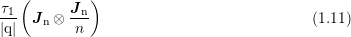in (1.7), and to neglect the contribution of velocity to the carrier energy, thus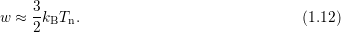This leads to a parabolic system of equations and is a very common approximation in today’s device simulators  . The two assumptions (1.11) and (1.12) can also be justified from a mathematical point of view by a scaling argument for vanishing Knudsen number  , which in addition shows that the time derivatives in the flux equations vanish. This leads to the energy transport model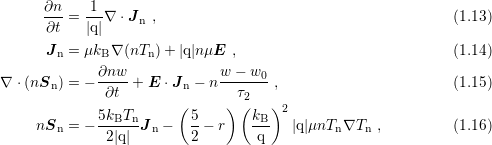where (1.10) has been used. As the model consists of two conservation equations and two constitutive equations, the name is slightly misleading.

The closure based on Fourier’s law can be improved by taking the third moment of the BTE into account  . A closure condition for the fourth-order tensor is obtained by assuming a heated Maxwellian distribution, leading to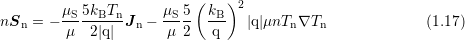instead of (1.16). This improved model based on four moments of the BTE is referred to as the four moments energy transport model. A comparison of (1.16) and (1.17) reveals an inconsistency of the energy transport model based on three moments, because the scalar prefactors in (1.16) are given by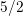and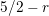, which means that the heat flux can be adjusted independently  .

#### 1.1.4 The Six-Moments Model

With the drift-diffusion model based on two moments of the BTE, the hydrodynamic model based on three moments and the energy transport model in modified form based on four moments of the BTE, it is tempting to construct more accurate models based on higher moments. However, the selection of suitable closure conditions becomes increasingly difficult. A model loosely based on six moments of the BTE has been proposed by Sonoda et al.  . A rigorous derivation of a model based on six moments has been carried out by Grasser et al.   in the diffusion limit of vanishing Knudson number: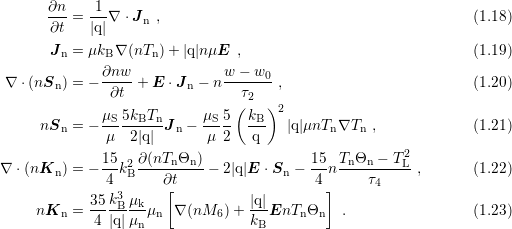The additional unknown variables are the second order temperature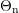and the kurtosis flux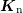. The parameter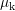can be related to a mobility for kurtosis and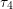is a macroscopic relaxation time for the fourth moment of the scattering operator. A closure condition for the sixth moment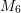is obtained via the empirical relation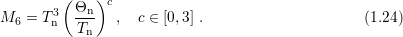A value of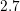for the parameterproved to provide highest accuracy when compared to Monte Carlo results and further shows higher numerical stability than other choices. Particularly, the Newton procedure for the choice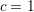has been reported to result in failure of convergence in most cases.

#### 1.1.5 The Monte Carlo Method

The macroscopic transport models presented so far are specified by a system of partial differential equations which approximate certain features of the BTE, for which suitable deterministic numerical solutions are sought. A stochastic approach for the solution of the full BTE is the Monte Carlo method, where the dynamics are simulated at the particle level   and which was already used in the 1960s for semiconductor device simulations (e.g.  ). The free streaming operator of the BTE is obtained from Newton’s laws of motion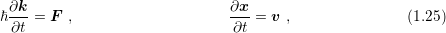while the scattering operator models instantaneous changes of the momentum of each particle in the device. Denote with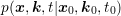the conditional probability of finding a particle at locationwith wave vectorat timegiven that the particle was at location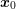with wave vector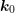at time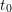. Since not only the distribution function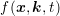, but also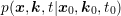satisfies the BTE, a formal integration of the BTE after merging the gradients with respect toandinto a joint gradient for the variable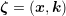yields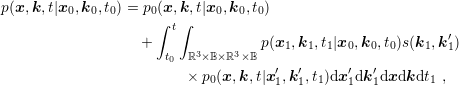(1.26)

where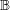denotes the Brillouin zone and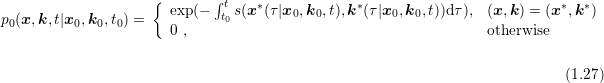is the conditional probability of finding a particle in state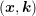at timegiven that the particle was located at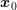with wave vector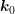at time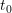without being scattered.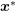and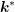are shorthand notations for the location of the particle at a given instance in time for a given initial position. Eq. (1.26) is an integral equation for, and the conjugate form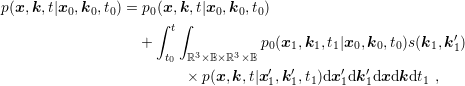(1.28)

is the basis for the usual Monte Carlo algorithm.

The Monte Carlo procedure uses a large sample of particles and simulates their dynamic behavior based on the integral formulation (1.28). To this end, the time intervals between the scattering events of each particle are chosen randomly based on the scattering operator, and particles propagate in collision-less flight according to (1.25) between scattering events. Macroscopic quantities such as average carrier energies are computed by suitable averages over the particle ensemble. In order to keep stochastic fluctuations reasonably small, a large number of particles is required, where the accuracy is asymptotically proportional to the square-root of the execution time unless special enhancement techniques for selected target quantities are applied  . While the Monte Carlo method provides a very high accuracy due to the simulation of particle kinetics and high flexibility with respect to the inclusion of additional physical details, it is computationally extremely expensive.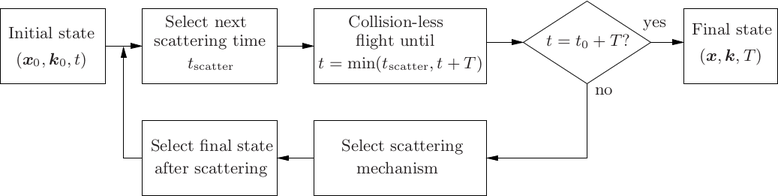Figure 1.1: Flowchart for the Monte Carlo method for a simulation from timeto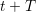.

### 1.2 Requirements of Modern TCAD

The various transport models and solution approaches to the BTE discussed in the previous section represent only a selection of the most popular methods for the simulation of charge transport in semiconductors. Regarding their accuracy and computational cost, these models typically range from the computationally cheap drift-diffusion model, which fails to reflect physics properly in the deca-nanometer regime, to the accurate Monte Carlo method, which has the drawback of extremely high computational costs. In the following, a discussion of the requirements of a state-of-the-art semiconductor device simulator is given. The individual requirements slightly overlap, but they should aid the reader in judging the importance of the proposed extensions of the deterministic Boltzmann solution method given in the remainder of this thesis.

#### 1.2.1 Accuracy

The need for a better reflection of the underlying physical processes compared to the drift-diffusion model has lead to the development of higher-order transport models such as the hydrodynamic model. As device dimensions decrease, ballistic effects become significant, which cannot be captured in the diffusion-limited regime of higher-order transport models  . As a consequence, it is desireable to have numerical methods which are computationally less expensive than the Monte Carlo method, but are able to resolve ballistic transport effects. Even though not covered by the semiclassical approach, quantum effects become increasingly important and should be reflected in the model.

#### 1.2.2 Charge Conservation

Kirchhoff’s current law states the conservation of charge. Consequently, charge conservation has to be ensured during the simulation of a single semiconductor device as well. The use of the box integration scheme for the drift-diffusion model guarantees charge conservation at the algebraic level. Similarly, charge conservation is provided for other moment-based methods. Moreover, the particle approach of the Monte Carlo method allows for charge conservation without difficulties. As a consequence, new simulation approaches need to ensure charge conservation in order to be competitive with existing methods.

#### 1.2.3 Self-Consistency

The electrostatic potential and the space charge are linked by the Poisson equation, which is a direct implication of Gauss’ Law. Since it is in general neither possible to explicitly express the potential as a function of the carrier densities, nor to express the carrier densities as an explicit function of the electrostatic potential only, any transport model used for charge transport is required to be solved self-consistently with the Poisson equation.

#### 1.2.4 Resolution of Complicated Domains

With the on-going miniaturization of semiconductor devices, inherent small variations in the fabrication process become significant with respect to the characteristic device lengths. Consequently, device geometries are no longer simple compositions of geometric primitives, but should be taken from topography simulations. Therein, complicated device geometries show up in a natural way, particularly for fully three-dimensional device layouts.

#### 1.2.5 Computational Feasibility

The incredible pace of semiconductor device technology requires that simulation results are available within a short time frame. In particular, simulation times in the range of weeks or even months are clearly not acceptable, because during that time technology has progressed and simulation results may be deprecated already before they are available. This is the main reason why Monte Carlo methods are – despite their high accuracy – not commonly used for every-day TCAD purposes.

#### 1.2.6 Extendibility

As devices are scaled down, formerly negligible physical effects become relevant and need to be considered in the simulation. Thus, it is desirable to use numerical methods which allow for such an inclusion without a large redesign of the whole simulator. For instance, boundary element methods based on the knowledge of the Green’s function of the underlying partial differential equations become invalid and eventually infeasible as soon as details such as e.g. space-dependent diffusion coefficients are demanded.

### 1.3 Outline

The remainder of this thesis focuses on a rather new approach based on a spherical harmonics expansion of the distribution function. The method is expected to yield the accuracy of Monte Carlo methods without the inherent disadvantages of stochastic models. Other approaches for the deterministic numerical solution of the BTE have been proposed, but they are considered to be limited to at most two-dimensional device geometries either because the full momentum space needs to be discretized  , or because the discretization method leads to nonlocal couplings resulting in huge memory requirements for the resulting linear system of equations  .

In Chap. 2 an introduction to the SHE method is given and the state-of-the-art for the method is presented, excluding the extensions proposed in this work. The chapter closes with a discussion of the compatibility of the SHE method with respect to modern TCAD.

Chap. 3 details the underlying physical processes and their consideration within the SHE method. A method for the inclusion of carrier-carrier scattering is proposed at the end of the chapter.

The mathematical structure of the SHE equations is investigated in detail in Chap. 4. A sparse coupling property of the SHE equations is shown for spherical energy bands. These coupling investigations then lead to a system matrix compression scheme, which allows for a memory efficient representation of the system matrix at high expansion orders.

Chap. 5 presents the extension of the SHE method to unstructured grids. All the required implementation details for the construction of suitable meshes are given.

In order to obtain the accuracy of higher-order expansions at lower computational effort, adaptive variable-order expansions are proposed and evaluated in Chap. 6. The possible savings mostly stem from the fact that on the one hand the distribution function is still close to equilibrium in inactive device regions, and on the other hand from the asymptotically exponential decay of the distribution function with respect to kinetic energy, hence lower resolution at higher energies is sufficient.

Recent developments in computing architecture are addressed in Chap. 7, where a parallel scheme for the convergence enhancement of the iterative solvers by means of preconditioners is suggested. It enables the full utilization of multi-core central processing units as well as the efficient use with massively parallel architectures such as graphics processing units.

The suggestions from Chaps. 4 to 7 are combined for the simulation of a MOSFET and a FinFET in Chap. 8. It is demonstrated that the proposed techniques blend well with each other and render the SHE method very attractive for modern TCAD.

An outlook to further possible improvements of the SHE method is presented in Chap. 9. A conclusion finally closes this thesis.